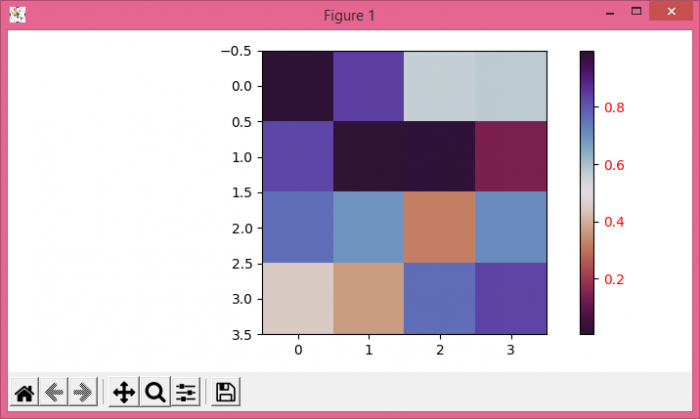# How to change the color of the ticks in the colorbar in Matplotlib?

To change the color of the ticks in the colorbar in matplotlib, we can take the following steps−

• Create a random 2D−Array using numpy, with 4☓4 dimension.
• Use imshow() method to display the data as an image.
• Create a colorbar using colorbar() method with scalar mappable instance of imshow().
• Use getp() method to return the value of an object's property or print all of them.
• Set the property of an artist object.
• To display the figure, use show() method.

## Example

import numpy as np
from matplotlib import pyplot as plt
plt.rcParams["figure.figsize"] = [7.00, 3.50]
plt.rcParams["figure.autolayout"] = True
data = np.random.rand(4, 4)
im = plt.imshow(data, cmap="twilight_shifted_r")
cbar = plt.colorbar(im)
cbar_yticks = plt.getp(cbar.ax.axes, 'yticklabels')
plt.setp(cbar_yticks, color='r')
plt.show()

## Output